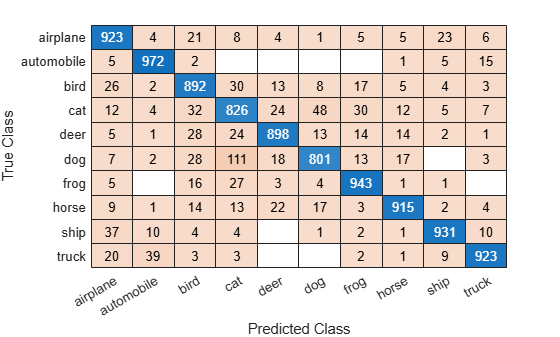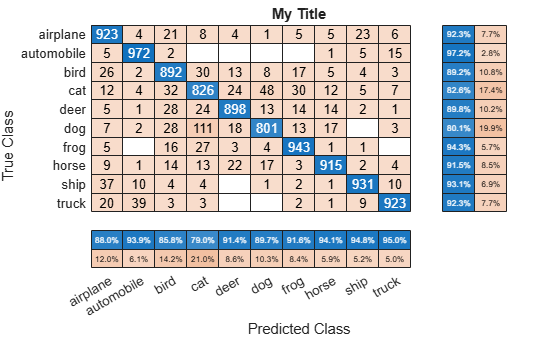# confusionmat

Compute confusion matrix for classification problem

## Syntax

``C = confusionmat(group,grouphat)``
``C = confusionmat(group,grouphat,'Order',grouporder)``
``[C,order] = confusionmat(___)``

## Description

example

````C = confusionmat(group,grouphat)` returns the confusion matrix `C` determined by the known and predicted groups in `group` and `grouphat`, respectively. ```
````C = confusionmat(group,grouphat,'Order',grouporder)` uses `grouporder` to order the rows and columns of `C`. ```

example

````[C,order] = confusionmat(___)` also returns the order of the rows and columns of `C` in the variable `order` using any of the input arguments in previous syntaxes.```

## Examples

collapse all

Load a sample of predicted and true labels for a classification problem. `trueLabels` are the true labels for an image classification problem and `predictedLabels` are the predictions of a convolutional neural network.

`load('Cifar10Labels.mat','trueLabels','predictedLabels');`

Calculate the numeric confusion matrix. `order` is the order of the classes in the confusion matrix.

`[m,order] = confusionmat(trueLabels,predictedLabels)`
```m = 10×10 923 4 21 8 4 1 5 5 23 6 5 972 2 0 0 0 0 1 5 15 26 2 892 30 13 8 17 5 4 3 12 4 32 826 24 48 30 12 5 7 5 1 28 24 898 13 14 14 2 1 7 2 28 111 18 801 13 17 0 3 5 0 16 27 3 4 943 1 1 0 9 1 14 13 22 17 3 915 2 4 37 10 4 4 0 1 2 1 931 10 20 39 3 3 0 0 2 1 9 923 ```
```order = 10x1 categorical airplane automobile bird cat deer dog frog horse ship truck ```

You can use `confusionchart` to plot a the confusion matrix as a confusion matrix chart.

```figure cm = confusionchart(m,order);```You do not need to calculate the confusion matrix first and then plot it. Instead, plot a confusion matrix chart directly from the true and predicted labels. You can also add column and row summaries and a title.

```figure cm = confusionchart(trueLabels,predictedLabels, ... 'Title','My Title', ... 'RowSummary','row-normalized', ... 'ColumnSummary','column-normalized');```The `ConfusionMatrixChart` object stores the numeric confusion matrix in the `NormalizedValues` property and classes in the `ClassLabels` property.

`cm.NormalizedValues`
```ans = 10×10 923 4 21 8 4 1 5 5 23 6 5 972 2 0 0 0 0 1 5 15 26 2 892 30 13 8 17 5 4 3 12 4 32 826 24 48 30 12 5 7 5 1 28 24 898 13 14 14 2 1 7 2 28 111 18 801 13 17 0 3 5 0 16 27 3 4 943 1 1 0 9 1 14 13 22 17 3 915 2 4 37 10 4 4 0 1 2 1 931 10 20 39 3 3 0 0 2 1 9 923 ```
`cm.ClassLabels`
```ans = 10x1 categorical airplane automobile bird cat deer dog frog horse ship truck ```

## Input Arguments

collapse all

Known groups for categorizing observations, specified as a numeric vector, logical vector, character array, string array, cell array of character vectors, or categorical vector.

`group` is a grouping variable of the same type as `grouphat`. The `group` argument must have the same number of observations as `grouphat`, as described in Grouping Variables (Statistics and Machine Learning Toolbox). The `confusionmat` function treats character arrays and string arrays as cell arrays of character vectors. Additionally, `confusionmat` treats `NaN`, empty, and `'undefined'` values in `group` as missing values and does not count them as distinct groups or categories.

Example: `{'Male','Female','Female','Male','Female'}`

Data Types: `single` | `double` | `logical` | `char` | `string` | `cell` | `categorical`

Predicted groups for categorizing observations, specified as a numeric vector, logical vector, character array, string array, cell array of character vectors, or categorical vector.

`grouphat` is a grouping variable of the same type as `group`. The `grouphat` argument must have the same number of observations as `group`, as described in Grouping Variables (Statistics and Machine Learning Toolbox). The `confusionmat` function treats character arrays and string arrays as cell arrays of character vectors. Additionally, `confusionmat` treats `NaN`, empty, and `'undefined'` values in `grouphat` as missing values and does not count them as distinct groups or categories.

Example: `[1 0 0 1 0]`

Data Types: `single` | `double` | `logical` | `char` | `string` | `cell` | `categorical`

Group order, specified as a numeric vector, logical vector, character array, string array, cell array of character vectors, or categorical vector.

`grouporder` is a grouping variable containing all the distinct elements in `group` and `grouphat`. Specify `grouporder` to define the order of the rows and columns of `C`. If `grouporder` contains elements that are not in `group` or `grouphat`, the corresponding entries in `C` are `0`.

By default, the group order depends on the data type of ```s = [group;grouphat]```:

• For numeric and logical vectors, the order is the sorted order of `s`.

• For categorical vectors, the order is the order returned by `categories(s)`.

• For other data types, the order is the order of first appearance in `s`.

Example: `'order',{'setosa','versicolor','virginica'}`

Data Types: `single` | `double` | `logical` | `char` | `string` | `cell` | `categorical`

## Output Arguments

collapse all

Confusion matrix, returned as a square matrix with size equal to the total number of distinct elements in the `group` and `grouphat` arguments. `C(i,j)` is the count of observations known to be in group `i` but predicted to be in group `j`.

The rows and columns of `C` have identical ordering of the same group indices. By default, the group order depends on the data type of `s = [group;grouphat]`:

• For numeric and logical vectors, the order is the sorted order of `s`.

• For categorical vectors, the order is the order returned by `categories(s)`.

• For other data types, the order is the order of first appearance in `s`.

To change the order, specify `grouporder`,

The `confusionmat` function treats `NaN`, empty, and `'undefined'` values in the grouping variables as missing values and does not include them in the rows and columns of `C`.

Order of rows and columns in `C`, returned as a numeric vector, logical vector, categorical vector, or cell array of character vectors. If `group` and `grouphat` are character arrays, string arrays, or cell arrays of character vectors, then the variable `order` is a cell array of character vectors. Otherwise, `order` is of the same type as `group` and `grouphat`.

## Alternative Functionality

• Use `confusionchart` to calculate and plot a confusion matrix. Additionally, `confusionchart` displays summary statistics about your data and sorts the classes of the confusion matrix according to the class-wise precision (positive predictive value), class-wise recall (true positive rate), or total number of correctly classified observations.